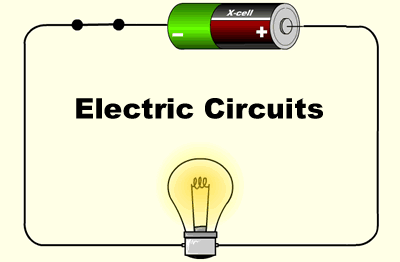# Electrical Circuits Quiz

MCQs on Electrical Circuits

31
Created on ByElectrical NotebookElectrical Circuits Quiz

1 / 11

1. Magnetic lines of forces –

2 / 11

2. Battery is a

3 / 11

3. For a sinusoidal AC voltage, peak factor or amplitude fator or crest factor is –

4 / 11

4. RMS value can be defined based on which of the following? –

5 / 11

5. An alternating voltage is given by, V = 10 sin 2πt. The frequency of the AC signal is –

6 / 11

6. In series R-L circuit power factor can be defined as –

7 / 11

7. If the length of a wire is doubled and diameter is halved, then the resistance will become _________ of the previous.

8 / 11

8. Calculate the value of energy stored in an inductor if the value of inductance is 10H and 2A of current flows through it.-

9 / 11

9. Frequency of Direct Current is

10 / 11

10. If a capacitor is connected with DC supply, it will act as –

11 / 11

11. Three lamps are connected in parallel across the voltage source. What happen if one lamp will burn out?